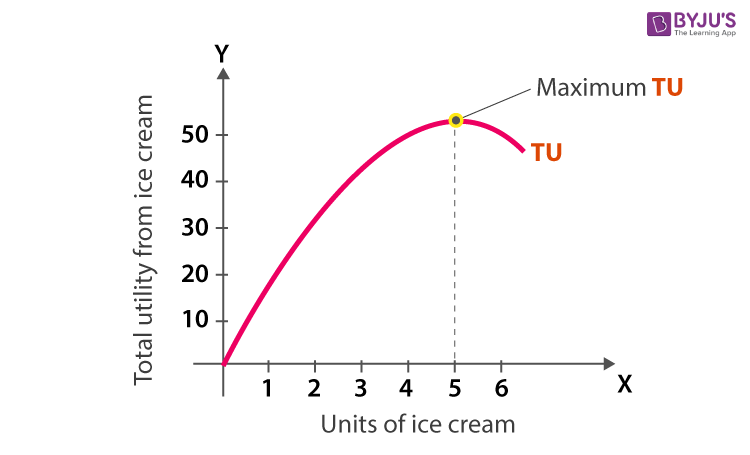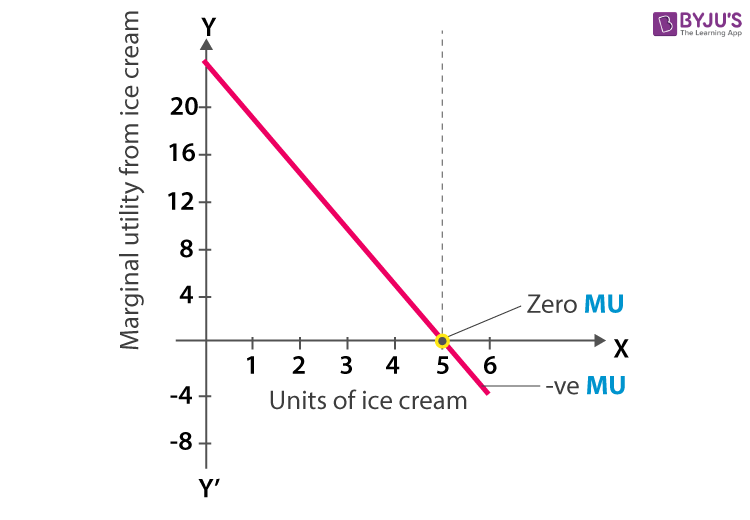# Sandeep Garg Macroeconomics Class 12: Chapter 2 Consumer’s Equilibrium

Sandeep Garg Class 12 Microeconomics Solutions Chapter 2 Consumer’s Equilibrium is explained by the expert Economics teachers from the latest edition of Sandeep Garg Microeconomics Class 12 textbook solutions. We at BYJU’S provide Sandeep Garg economics class 12 Solutions to give comprehensive insight about the subject to the students. These insights help as a priceless benefit to students while completing their homework or while studying for their exams. There are numerous concepts in economics, however, we at BYJU’S provide the students with the solution from Consumer’s Equilibrium, which will be useful for the students to score well in the board examinations.

## Sandeep Garg Solutions Class 12 – Chapter 2 – Part A – Microeconomics

Question 1

Define Total Utility.

Ans: Total Utility refers to the total satisfaction obtained from the consumption of all possible units of a commodity.

Question 2

Explain how the Total Utility and Marginal Utility are calculated, by using graphical representation.

 Ice Creams consumed Marginal Utility (MU) Total Utility (TU) 1 20 20 2 16 36 3 10 46 4 4 50 5 0 50 6 -6 44

Solution:Question 3

Explain the Law of Diminishing Utility.

Ans: The Law of Diminishing Utility (LDMU) states that as we consume more and more units of a commodity, the utility derived from each successive unit goes on decreasing.

Question 4

Mention 4 Assumptions of Law of Diminishing Utility.

Ans: The 4 assumptions of Law of Diminishing Utility are:

• Rational Consumer
• Perfect Knowledge
• Fixed Income and prices
• Independent utilities

Question 5

What is Indifference Curve?

Ans: Indifference Curve refers to the graphical representation of various alternatives combinations of bundles of 2 goods among which the consumer is indifferent.

Question 6

Expand MRS.

Ans: MRS stands for Marginal Rate of Substitution.

Question 7

Give 3 Assumptions of Indifference Curve.

Ans: The three assumptions of indifference curve are :

• Non-satiety
• 2 commodities
• Ordinal Utility

The above-provided solutions are considered to be the best solution for ‘Sandeep Garg Microeconomics Class 12 Solutions Chapter 2 Consumer’s Equilibrium’. Stay tuned to BYJU’S to learn more.